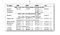# CowMask — Data Augmentation for Self-Supervised Models

## Google Research recently published CowMask — a new SOTA masking-based augmentation method. The method allows training more stable self-supervised models.Results on CIFAR-100 test set, error rates as mean ± stdev of 5 independent runs. Source: Arxiv

# Consistency in semi-supervised training

Consistency regularization is a semi-supervised training technique that trains a model using a small amount of labeled data. It works in such a way that the model is trained to resist changes in unlabeled data.

The researchers adapted the Mean Teacher framework and used it as a base for the approach. They used two networks: a student network and a teacher network. Both models predict vectors with probabilities for each class. The student network is trained in a standard manner using gradient descent. After each update of the parameters, the weights of the teacher network are updated so that they are an exponential moving average of the weights of the student model. At the same time, there is a hyperparameter with the help of which the stability and speed of how the teacher network follows the student network are regulated.

Written by